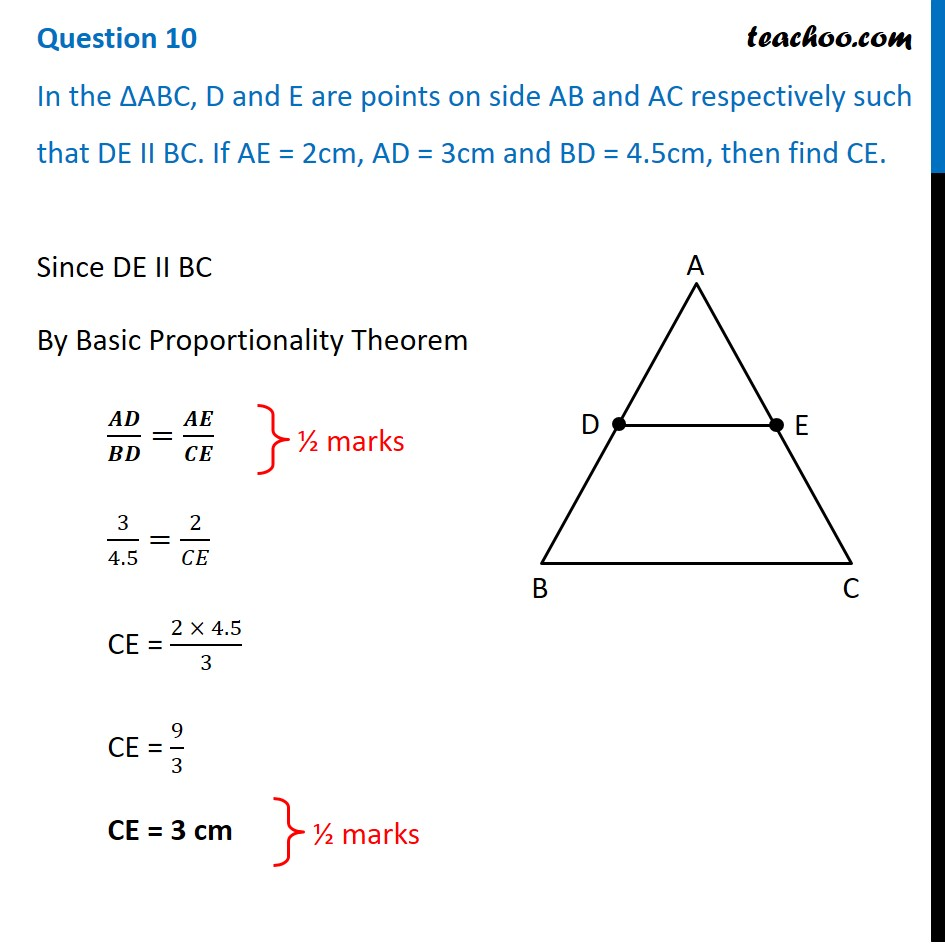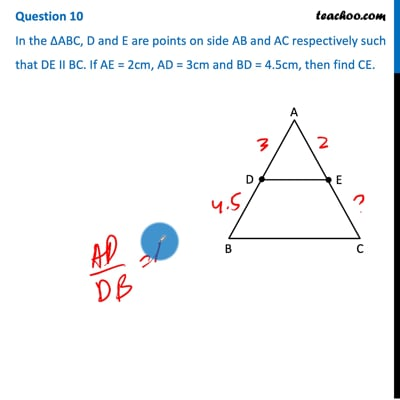CBSE Class 10 Sample Paper for 2021 Boards - Maths Standard

Class 10
Solutions of Sample Papers for Class 10 Boards

## In the ∆ABC, D and E are points on side AB and AC respectively such that DE II BC. If AE = 2cm, AD = 3cm and BD = 4.5cm, then find CE.This video is only available for Teachoo black users

Introducing your new favourite teacher - Teachoo Black, at only ₹83 per month

### Transcript

Question 10 In the ∆ABC, D and E are points on side AB and AC respectively such that DE II BC. If AE = 2cm, AD = 3cm and BD = 4.5cm, then find CE.Since DE II BC By Basic Proportionality Theorem 𝑨𝑫/𝑩𝑫=𝑨𝑬/𝑪𝑬 3/4.5=2/𝐶𝐸 CE = (2 × 4.5)/3 CE = 9/3 CE = 3 cm